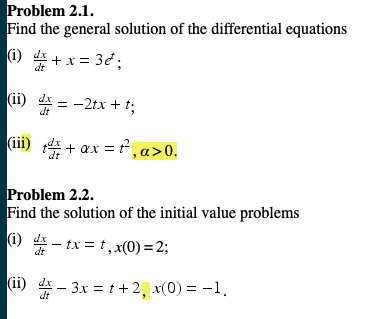Problem 2.1.Find the general solution of the differential equations1) dr(ii)些--2tx + t;dtProblem 2.2.Find the solution of the initial value problems(i) T-tx=t,x(0)=2;(i) 4- 3x -+2x(0)1dt

Question

problem 2.1 iii and 2.2 iihelp_outlineImage TranscriptioncloseProblem 2.1. Find the general solution of the differential equations 1) dr (ii) 些--2tx + t; dt Problem 2.2. Find the solution of the initial value problems (i) T-tx=t,x(0)=2; (i) 4- 3x -+2x(0)1 dt fullscreen
Step 1

Problem 2.1 (iii)

Given ODE can be transformed into a Linear ODE

Step 2

Procedure for solving Linear differential equation:

Step 3

From the given problem, we get the ...

Want to see the full answer?

See Solution

Want to see this answer and more?

Our solutions are written by experts, many with advanced degrees, and available 24/7

See Solution
Tagged in

Calculus